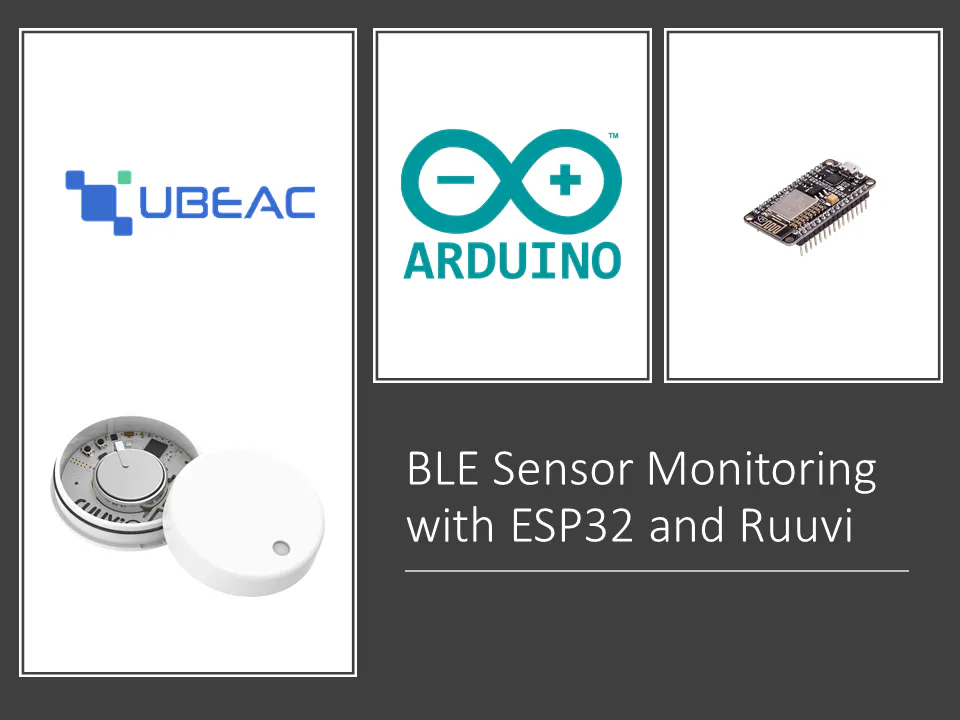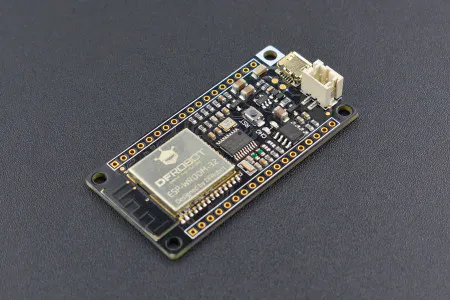Published © MIT

# BLE Weather Station with ESP32 and Ruuvi

Using just an ESP32 and a RuuviTag, monitor the temperature, humidity, and other environmental sensors on one single IoT platform!

IntermediateFull instructions provided1 hour2,740## Things used in this project

### Hardware componentsDFRobot FireBeetle ESP32 IOT Microcontroller (Supports Wi-Fi & Bluetooth)
×1
 RuuviTag
×1

### Software apps and online servicesuBeac IoT Platform Free SubscriptionArduino IDE

Read more

## Code

### BLE Scan RuuviTag

Arduino
```#include <BLEDevice.h>
#include <BLEUtils.h>
#include <BLEScan.h>
#include <BLEAdvertisedDevice.h>
#include <BLEAddress.h>
#include <WiFi.h>
#include <HTTPClient.h>

int scanTime = 5; //In seconds
BLEScan* pBLEScan;
int temp, hum, pressure, ax, ay, az, voltage_power, voltage, power, rssi_ruuvi, movement, measurement;
String payload;
String MAC_add = "MAC ADDRESS HERE"; //All the identified MAC addresses will go in one String

const char* ssid     = "WIFI SSID HERE";              //Main Router
const char* password = "WIFI PASSWORD HERE";            //Main Router Password
const char* url = "UBEAC GATEWAY URL HERE";

//Converts hexadecimal values to decimal values
int hexadecimalToDecimal(String hexVal)
{
int len = hexVal.length();
int base = 1;

int dec_val = 0;

for (int i = len - 1; i >= 0; i--)
{
if (hexVal[i] >= '0' && hexVal[i] <= '9')
{
dec_val += (hexVal[i] - 48) * base;

base = base * 16;
}
else if (hexVal[i] >= 'A' && hexVal[i] <= 'F')
{
dec_val += (hexVal[i] - 55) * base;

base = base * 16;
}
}
return dec_val;
}

//Decodes RUUVI raw data and arranges it in an array
void decodeRuuvi(String hex_data, int rssi){
if(hex_data.substring(4, 6) == "05"){
temp = hexadecimalToDecimal(hex_data.substring(6, 10))*0.005;
hum = hexadecimalToDecimal(hex_data.substring(10, 14))*0.0025;
pressure = hexadecimalToDecimal(hex_data.substring(14, 18))*1+50000;

ax = hexadecimalToDecimal(hex_data.substring(18, 22));
ay = hexadecimalToDecimal(hex_data.substring(22, 26));
az = hexadecimalToDecimal(hex_data.substring(26, 30));

if(ax > 0xF000){
ax = ax - (1 << 16);
}
if(ay > 0xF000){
ay = ay - (1 << 16);
}
if (az > 0xF000){
az = az - (1 << 16);
}

voltage_power = hexadecimalToDecimal(hex_data.substring(30, 34));
voltage = (int)((voltage_power & 0x0b1111111111100000) >> 5) + 1600;
power = (int)(voltage_power & 0x0b0000000000011111) - 40;

rssi_ruuvi = rssi;

movement = hexadecimalToDecimal(hex_data.substring(34, 36));
measurement = hexadecimalToDecimal(hex_data.substring(36, 40));
}
}

//Converts decoded RUUVI data into uBeac JSON format
void uBeacRuuvi(){
payload = "[{\"id\": \"MyRUUVI\", \"sensors\": [{\"id\": \"Temperature\", \"value\": \$temperature\$}, {\"id\": \"Humidity\", \"value\": \$humidity\$}, "
"{\"id\": \"Pressure\", \"value\": \$pressure\$}, {\"id\": \"Acceleration\", \"value\": {\"ax\": \$ax\$,\"ay\": \$ay\$,\"az\": \$az\$,}}, "
"{\"id\": \"Voltage Power\", \"value\": \$voltage_power\$}, {\"id\": \"Voltage\", \"value\": \$voltage\$}, {\"id\": \"Power\", \"value\": \$power\$}, "
"{\"id\": \"RSSI\", \"value\": \$rssi\$}, {\"id\": \"Movement Counter\", \"value\": \$movement\$}, {\"id\": \"Measurement Sequence\", \"value\": \$measurement\$}]}]";

payload.replace("\$temperature\$",String(temp));
payload.replace("\$humidity\$",String(hum));
payload.replace("\$pressure\$",String(pressure/100));
payload.replace("\$ax\$",String(ax*9.81/1000));
payload.replace("\$ay\$",String(ay*9.81/1000));
payload.replace("\$az\$",String(az*9.81/1000));
payload.replace("\$voltage_power\$",String(voltage_power));
payload.replace("\$voltage\$",String(voltage));
payload.replace("\$power\$",String(power));
payload.replace("\$rssi\$",String(rssi_ruuvi));
payload.replace("\$movement\$",String(movement));
payload.replace("\$measurement\$",String(measurement));
}

//Class that scans for BLE devices
class MyAdvertisedDeviceCallbacks: public BLEAdvertisedDeviceCallbacks {
void onResult(BLEAdvertisedDevice advertisedDevice) {
//Scans for specific BLE MAC addresses
if(MAC_add.indexOf(advertisedDevice.getAddress().toString().c_str()) >= 0){ //If the scanned MAC address is in the identified MAC address String
String raw_data = String(BLEUtils::buildHexData(nullptr, (uint8_t*)advertisedDevice.getManufacturerData().data(), advertisedDevice.getManufacturerData().length()));
raw_data.toUpperCase();
decodeRuuvi(raw_data, advertisedDevice.getRSSI());
uBeacRuuvi();
}

//  Sends RuuviTag JSON data to IoT clod platform
if(WiFi.status()== WL_CONNECTED){

HTTPClient http;

http.begin(url);
int httpResponseCode = http.POST(payload);

if(httpResponseCode>0){
String response = http.getString();
Serial.println(httpResponseCode);
}
http.end();

}else{
Serial.println("Error in WiFi connection");
}
}
};

void setup() {
Serial.begin(115200);

//Connect to Local WiFi
delay(4000);   //Delay needed before calling the WiFi.begin

WiFi.begin(ssid, password);

while (WiFi.status() != WL_CONNECTED) { //Check for the connection
delay(1000);
Serial.println("Connecting to WiFi..");
}

Serial.println("Connected to the WiFi network");

//BLE scanning
Serial.println("Scanning...");

BLEDevice::init("");
pBLEScan = BLEDevice::getScan(); //create new scan
pBLEScan->setAdvertisedDeviceCallbacks(new MyAdvertisedDeviceCallbacks());
pBLEScan->setActiveScan(true); //active scan uses more power, but get results faster
pBLEScan->setInterval(100);
pBLEScan->setWindow(99);  // less or equal setInterval value
}

void loop() {
BLEScanResults foundDevices = pBLEScan->start(scanTime, false);
pBLEScan->clearResults();   // delete results fromBLEScan buffer to release memory
delay(3000);
}
```

## Credits

### Amir Pournasserian

10 projects • 13 followers
Data scientist, machine learning specialist, IoT nerd. CEO of Momentaj and Founder of uBeac, IoT thinktanks.